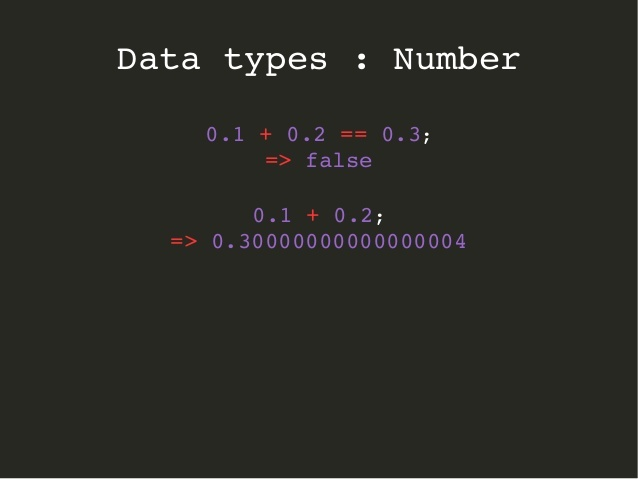Home » why 0.1 + 0.2 does not equal to 0.3?

# why 0.1 + 0.2 does not equal to 0.3?#### Have you ever discovered a scenario wherever you probably did some arithmetic computation on decimal numbers in a computer and it returned an unexpected weird result?

That one question you’d have asked is why 0.1 + 0.2 does not equal to 0.3?

##### Examples:
• 0.1 + 0.2 = 0.3 but computer says 0.30000000000000004
• 6 * 0.1 = 0.6 but computer says 0.6000000000000001
• 0.11 + 0.12 = 0.23 but again computer says 0.22999999999999998
• 0.1 + 0.7 = 0.7999999999999999
• 0.3+0.6 = 0.8999999999999999 and so on… There are several other such cases.

Note: It is also a interview questions that an interviewer asks “output of 0.1 + 0.2 == 0.3”? true or false? Simply to see how well you know arithmetic computations on decimal numbers by computer.

##### In straightforward sentences, the explanation behind this is:
• The computer uses base-2 floating-point number whereas Human(We) use base-10 floating-point numbers.
• In binary, decimal numbers cannot represent all decimals precisely and have infinite representations.
• So computers can not accurately represent numbers like 0.1, 0.2 or 0.3 at all as it uses binary floating-point format.
##### Now let’s understand the elaborated reason behind this:
• In base 10 system (Used by human/us), fractions can be expressed precisely if it uses a prime factor of the base (10).
• 2 and 5 are the prime factors of 10.
• 1/2, 1/4, 1/5 (0.2), 1/8, and 1/10 (0.1) can be expressed precisely as a result of denominators use prime factors of 10.
• Whereas, 1/3, 1/6, and 1/7 are repeating decimals as a result of denominators use a prime factor of 3 or 7.
• In base 2 (binary) system on the other hand (Used by computer), fractions can be expressed precisely if it uses a prime factor of the base (2).
• 2 is the only prime factor of 2.
• So 1/2, 1/4, 1/8 can all be expressed precisely because the denominators use prime factors of 2.
• Whereas 1/5 (0.2) or 1/10 (0.1) are repeating decimals.
• So we end up with leftovers for these repeating decimals and that carries over when we convert the computer’s base 2 number into a human-readable base 10 number.
##### Let’s take 0.1 as an example to understand binary and decimal representations:
• 0.1 is one-tenth(1/10) and to get binary representation (bicimal) of 0.1, we need to use the binary long division that is to divide binary 1 by binary 1010 (1/1010) like below:
• You can see above that the division process would never end and repeats forever with the digits in the quotient because 100 reappears as the dividend.
• so the binary representation of our decimal number 0.1 will be as below:
• Now above result can be slightly greater or Less than 0.1 which depends upon how many bits of precision are used.
• In half-precision which uses 11 significant bits, the floating-point approximation of 0.1 could be less than 0.1
```so 0.1 rounds to 0.0001100110011 in binary and 0.0999755859375 in decimal.

That's why the result becomes slightly less than 0.1
0.0999755859375 < 0.1```
• In double-precision floating-point which uses 53 bits, the floating-point approximation of 0.1 could be greater than 0.1
```so 0.1 rounds to 0.0001100110011001100110011001100110011001100110011001101 in binary
and 0.1000000000000000055511151231257827021181583404541015625 in decimal.

That's why the result becomes slightly greater than 0.1
0.1000000000000000055511151231257827021181583404541015625 > 0.1```

Due to all this, a decimal point may not give exact floating-point result as

• In pure math, every decimal has an equivalent bicimal (decimal converted to binary)
• In floating-point math, this is can be simply not true and that we do not get precise value for every decimal number.
Note: In most calculators, we see 0.1 + 0.2 = 0.3 despite the fact that that’s also a computing device. The explanation is that it uses additional guard digits to get around this downside by rounding off the final few bits.
##### References:

Below are some useful links you can refer to get even additional dipper into the floating-point arithmetics in computer.

I hope this can clear a number of your queries on why the computer does not return an expected output when we do the mathematical operation with decimal numbers.

Also, I believe you would be in a better position now to reply back if someone asks you why 0.1 + 0.2 does not equal to 0.3?

Happy Learning!!!